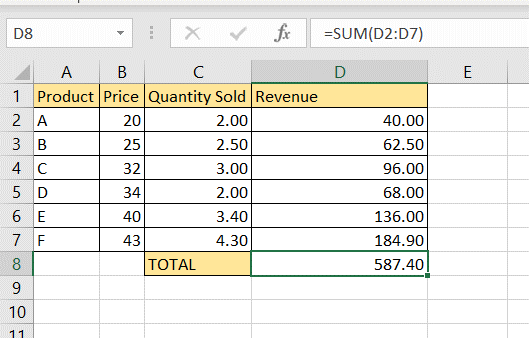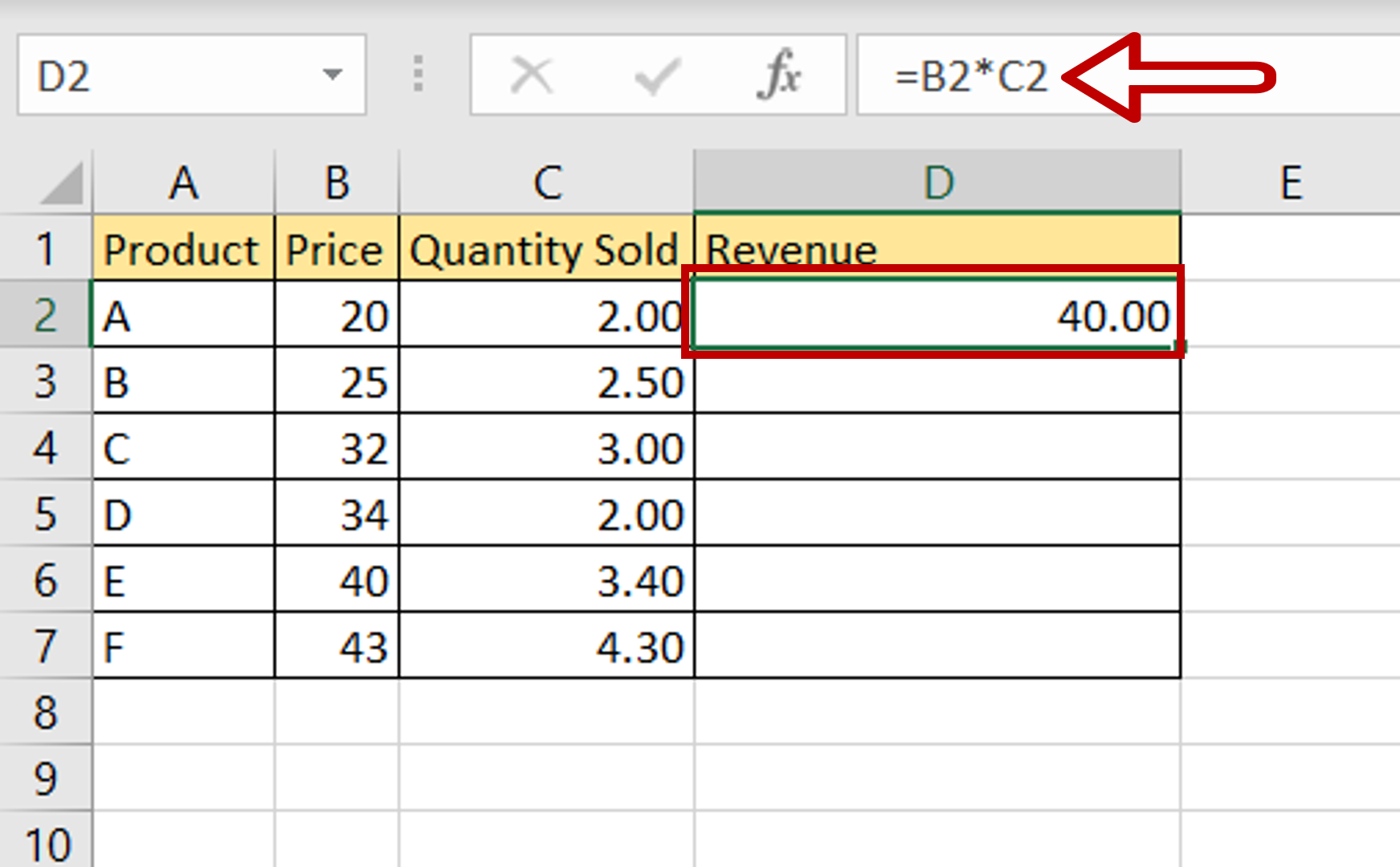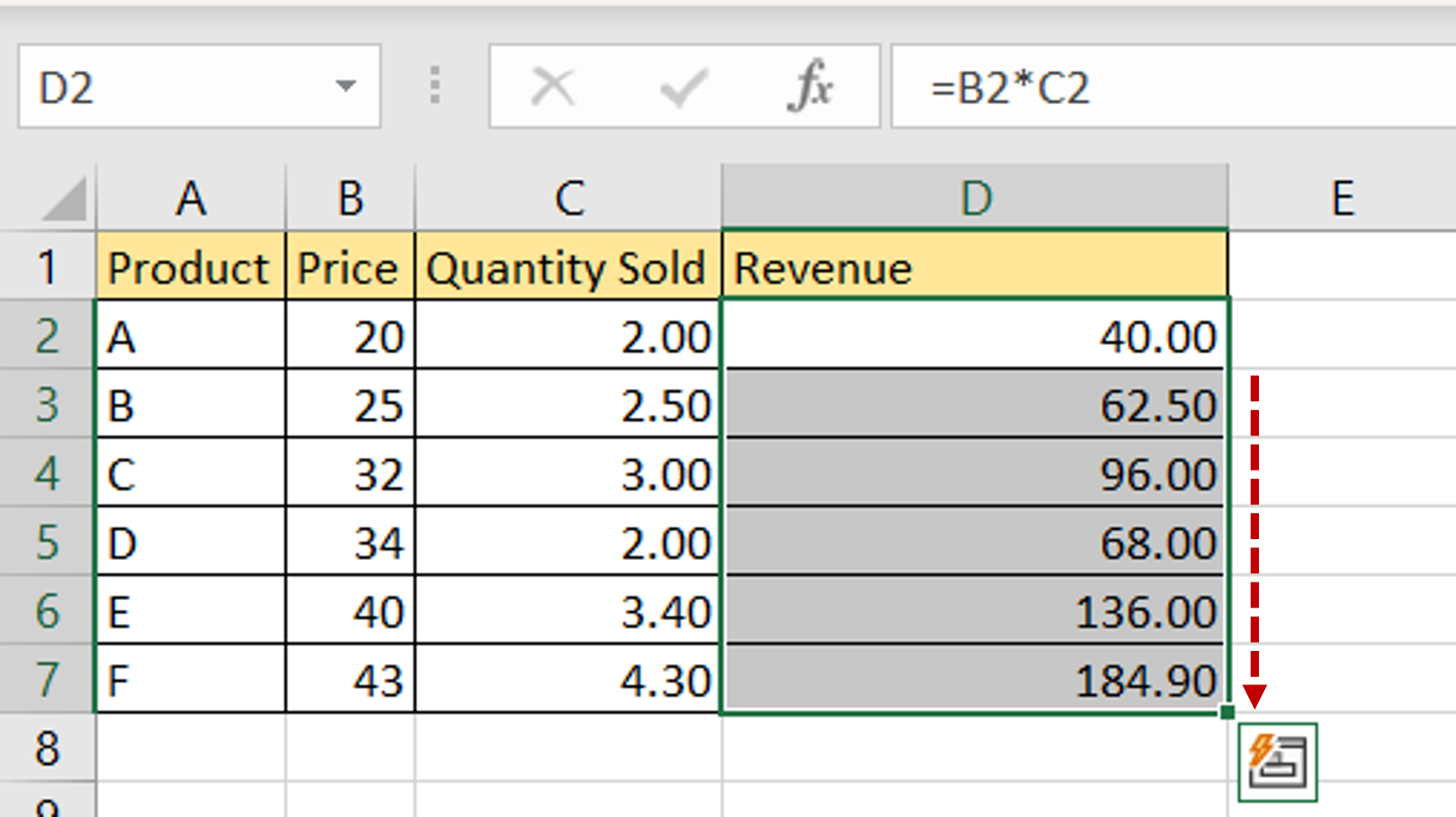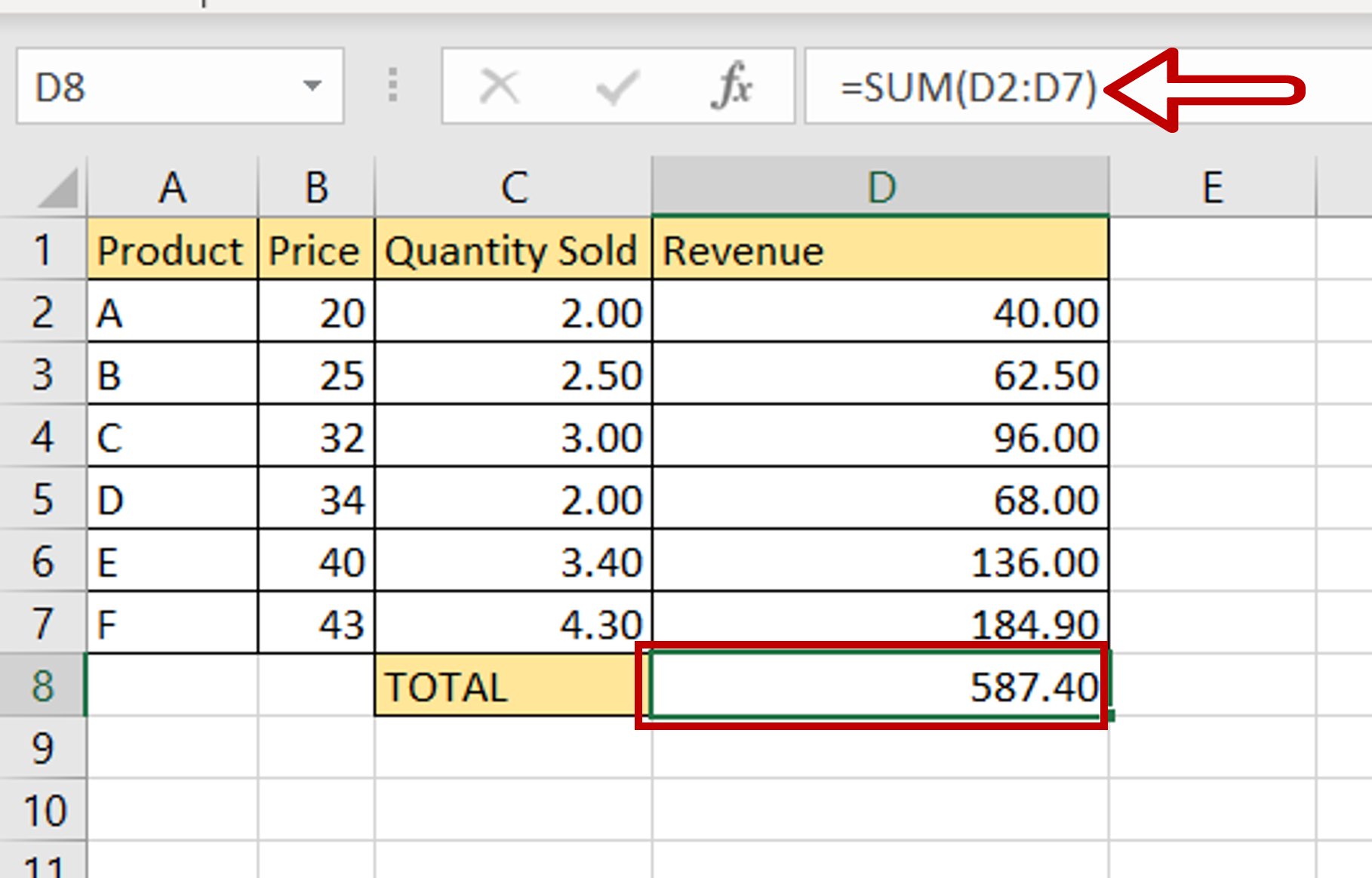# How to calculate total revenue in Excel

You can watch a video tutorial here.Excel is widely used for calculations due to the several mathematical operators and functions that it has. Many small businesses use Excel to keep track of their daily operations. Calculating revenue can be easily done in Excel using the quantity sold and the price. The formula is as follows:

Revenue = Price * Quantity Sold

### Step 1 – Enter the formula– Select the cell in which the result is to be displayed
– In the first cell of the ‘Revenue’ column, enter the formula using cell references:
=Price * Quantity Sold

### Step 2 – Copy the formula– Using the fill handle from the first cell, drag the formula to the remaining cells
OR
a) Select the cell with the formula and press Ctrl+C or choose Copy from the context menu (right-click)
b) Select the rest of the cells in the column and press Ctrl+V or choose Paste from the context menu (right-click)

### Step 3 – Calculate total revenue– Select the cell in which the total is to be displayed
– Type the formula using cell references:
=SUM(Revenue)
– Press Enter

### Step 4 – Check the result– The revenue from each product is displayed
– The total revenue amount is computed as well